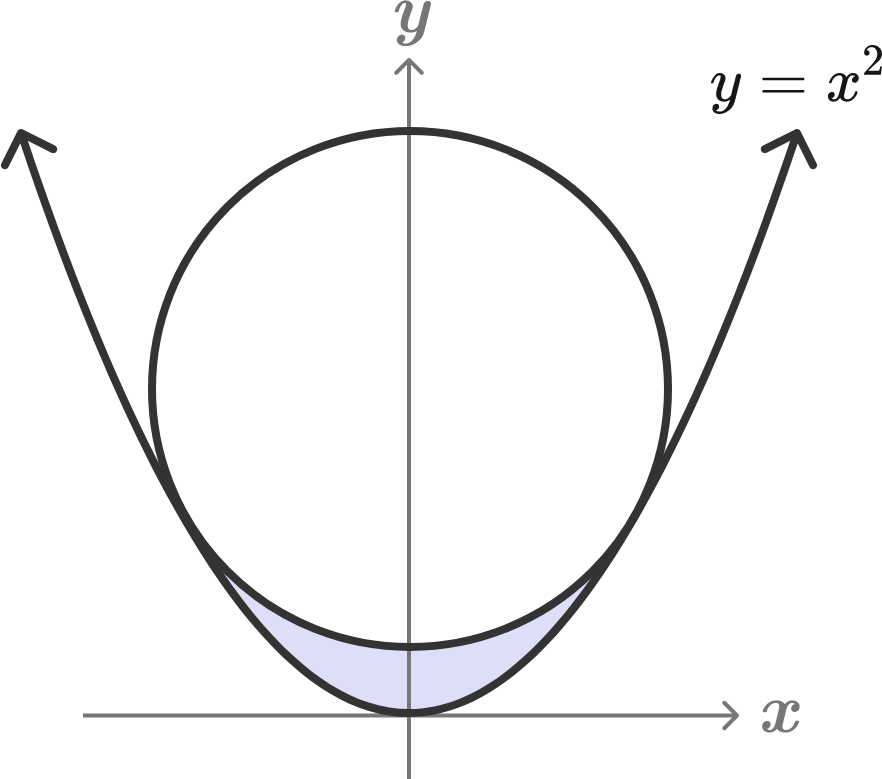# A Nice Geometry Problem (1)

Calculus Level 4A circle of radius 1 is tangent to the parabola $y=x^2,$ as shown.

Find the gray area between the circle and the parabola.

If the area can be written as $\dfrac{A\sqrt{A}}{B}-\dfrac{\pi}{A},$ then what is $A+B?$

×

Problem Loading...

Note Loading...

Set Loading...